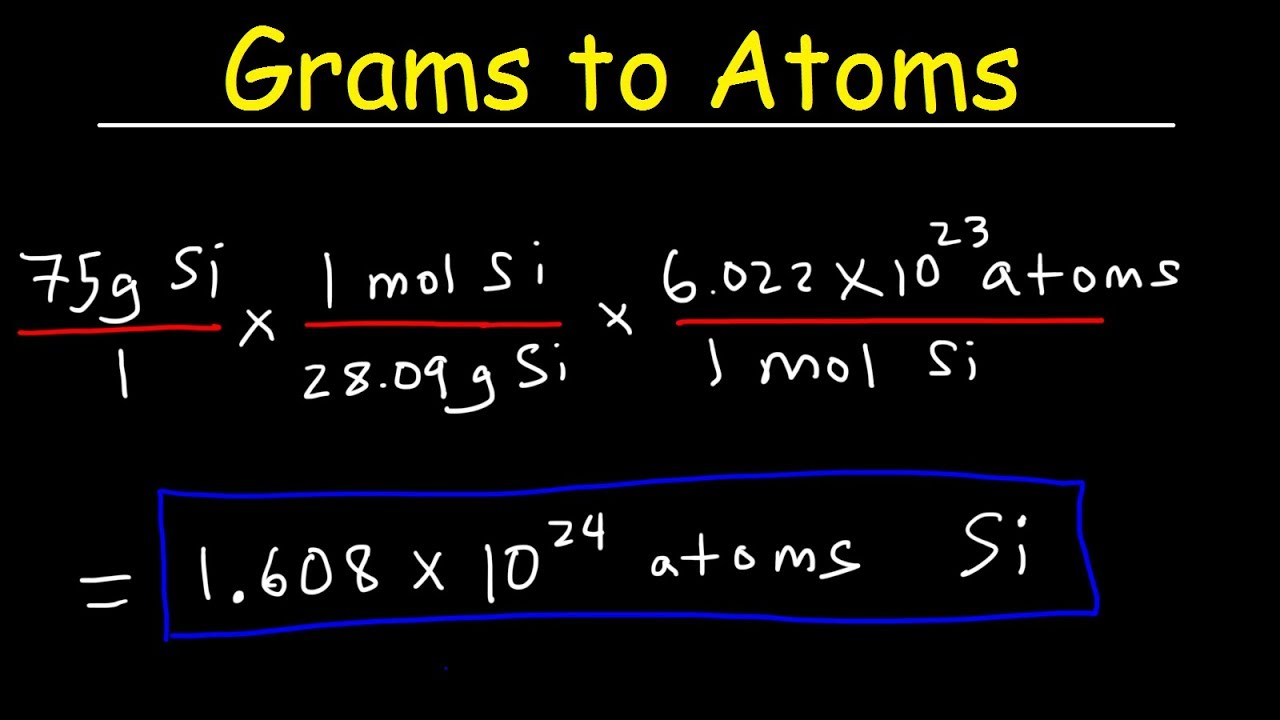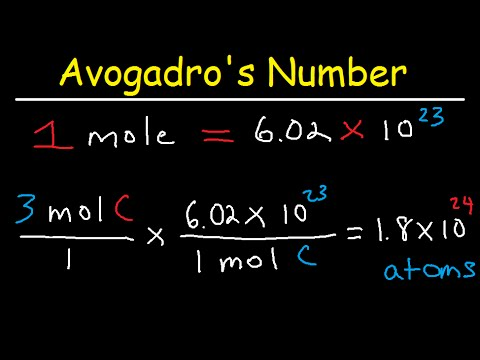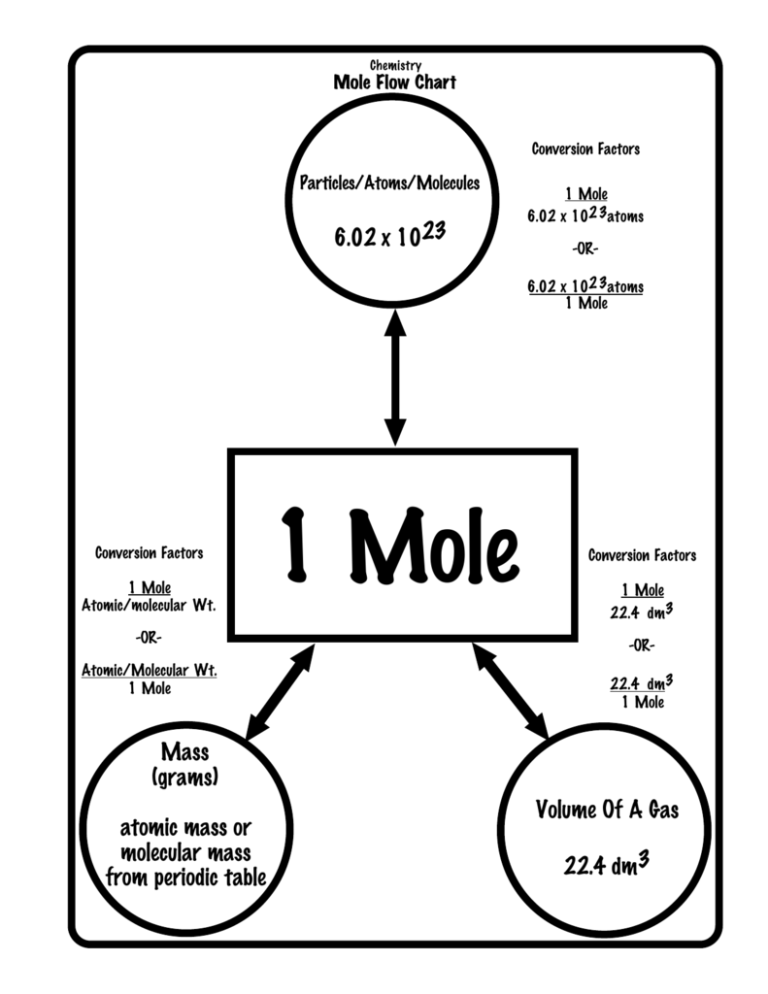# Grams to atoms calculator -step by step guide

Grams to Atoms Calculator is a free online tool that shows the particle’s conversion from grams to atoms. The online grams to atoms calculator tool speeds up the conversion by displaying the conversion to atoms in a fraction of a second. Thus, the grams to atoms calculator is a very useful tool.

Grams to Atoms Calculator uses a formula to calculate the mass of a substance in grams in relation to the number of atoms (or molecules) in the substance and the molar mass of that substance. You can use this calculator to find the missing parameter by entering the values of any two parameters in the input fields.

The Grams to Atoms Calculator makes it simple to determine the value of any of the three parameters in the preceding formula, provided we know the other two parameters.## Grams to atoms calculator with steps

The following is how to use the grams to atoms calculator:

In the first step, input the atomic mass number, grams, and x into the appropriate input fields. The grams to atoms calculator is a very useful tool.

Next, in the second step, to obtain the result, click the “Calculate x” button.

Finally, in the third step, in the output field, the conversion from grams to atoms will be displayed.

## Grams to atoms calculator chemistry

The grams to atoms calculator is a very useful tool.

We can use the following equation to calculate the particle conversion from grams to atoms:

So, assume you have ten grams of carbon. We should now convert it into atoms.

We know that 1 mole of carbon has a molar mass of 12. This translates to 12 grams per mole.

Furthermore, the Avogadro constant is 6.02 x 10^23.

As a result, we give the carbon conversion from grams to atoms as:

= 10(1/12)( 6.02 x 10^23)

= 5.02 x 10^23

As a result, 10 grams of carbon equals 5.02 x 10^23 atoms.

We can use the given formula below to calculate the number of atoms (or molecules) in a given sample of a substance:

Also, we calculate the number of atoms as NA * Substance mass (g) / Molar mass (g/mol), where NA is the Avogadro constant.

Again, we give the number of particles contained in one mole of a substance as the Avogadro constant NA = 6.0221407610^23 mol-1.

The Avogadro constant was calculated in such a way that the mass of one mole of any chemical compound in grams is arithmetically equal to the average mass of one atom (or molecule) of the given compound which is written in terms of daltons (universal atomic mass units).

We define one dalton as one-twelfth the mass of one carbon-12 atom, which is roughly equivalent to the mass of one nucleon. Moreover, a nucleon consists of proton and neutron.## Grams of gold to atoms calculator

How many moles of gold are there in one gram? The solution is 0.0050770041918285.

We’re assuming you’re converting between moles of gold and grams of gold.

Gold’s molecular formula is Au.

And, the mole is the SI base unit for substance amount.

1 mole is equal to 196.96655 grams of gold.

196.96655 grams = 196.96655 moles of gold

2 moles of gold = 393.9331 grams

3 moles of gold = 590.89965 grams

4 moles of gold = 787.8662 grams

5 moles of gold = 984.83275 grams

6 moles of gold = 1181.7993 grams

7 moles of gold = 1378.76585 grams

8 moles of gold = 1575.7324 grams

9 moles of gold = 1772.69895 grams

10 moles of gold = 1969.6655 grams

## Sodium atoms to grams calculator

How many moles of sodium are there in one gram? The solution is 0.043497607849056.

We’re assuming you’re converting moles of sodium to grams.

Sodium has the molecular formula Na.

The mole is the SI base unit for substance amount.

1 mole is equal to 22.98977 grams of sodium, or 1 mole.

one mole of sodium = 22.98977 grams of sodium

two moles of sodium = 45.97954 grams of sodium

three moles of sodium = 68.96931 grams of sodium

four moles of sodium = 91.95908 grams of sodium

five moles of sodium = 114.94885 grams of sodium

six moles of sodium = 137.93862 grams of sodium

seven moles of sodium = 160.92839 grams of sodium

eight moles of sodium = 183.91816 grams of sodium

nine moles of sodium = 206.90793 grams of sodium

ten moles of sodium = 229.8977 grams of sodium

## Atoms to grams calculator online

Grams to Atoms Calculator is a free of cost online tool that shows the particle’s conversion from grams to atoms. It also shows the particle’s conversion from atoms to grams.

Again, the calculator computes the substance’s mass in grams or quantity in moles. Moreover, it can function as a gram to moles calculator or a moles to grams calculator depending on the input data. It also displays the chemical substance’s molar mass and the details of its calculation for reference.

## How do you convert from atoms to grams

If you recognize the amount of particles in one mole and how to determine the amount of grams in one mole of that material, you can convert from atoms to grams.

Assume you have 3×10^24 chlorine atoms. How much chlorine is in that?

So, to begin, you must first convert from atoms to moles.

Also, you can use the molar mass to convert from mol to grams now that you know how many mol of chlorine 3×10^24 atoms is equal to.

Thus, this means that having 3×10^24 atoms of chlorine is equivalent to having 176.6 grams of chlorine.

What if you know how many atoms of an element you have, but the compound you’re talking about isn’t just that element? For example, suppose you know you have 5.55×10^23 atoms of oxygen but want to calculate the mass of CO2. How would you go about doing that?

Read Also: Temporal Isolation: Example & Definition

You are aware that each CO2 molecule contains two oxygen atoms. This means that for every oxygen atom, there is a complete CO2 molecule. We can use this conversion factor to calculate the mass of CO2.

So, look at this:

Thus, the only difference between what we had done previously and what we are doing now is the first step. You’ll need to figure out how many CO2 molecules there are:

You can now do exactly what you used to do!

You can calculate the grams of CO2 using only the number of oxygen atoms.

Always keep track of your units when converting from atoms to grams!## How to calculate number of atoms in grams

Scientists have listed the atomic weight of each element in the periodic table of elements. Also, scientists describe the mass of atoms using atomic mass units (amu), so think of atomic weights in terms of amus. Thus, we describe the number of atoms in a mole of an element by Avogadro’s constant, 6.02 x 10^23. Weighing a sample of an element yields the element’s mass in grams. You can also calculate the number of atoms in a sample if you have all three pieces of information: atomic weight, grams, and Avogadro’s number.

Therefore, to find the number of atoms in a sample, divide its weight in grams by the periodic table’s amu atomic mass, then multiply the result by Avogadro’s number: 6.02 x 10^23.

The volume of a gas at a given pressure and temperature is proportional to the number of molecules or atoms, regardless of gas type, according to Avogadro’s original theory.

We credit him with the concept, despite the fact that he did not determine the exact proportion.

On a human scale, Avogadro’s number is the ratio of atomic mass to physical mass. So, we define the number of elementary particles (atoms, molecules, compounds, etc.) per mole of a substance as Avogadro’s number. In addition to this, it is denoted by the symbol NA and is equal to 6.022 x 10^23 mol^-1.

Avogadro’s number is roughly equivalent to a dozen or so. A dozen molecules are equivalent to 12 molecules. A gross contains 144 molecules. Also, the value of Avogadro’s number is 6.022 x 10^23 molecules. Scientists can compare and discuss large numbers using Avogadro’s number, which is useful because everyday substances contain many atoms and molecules.## What is a mole?

The mole, generally written as mol, is the SI unit of measurement for the quantity of a “chemical entity” like electrons or protons. We define the amount of particles in a substance containing 12 grams of pure carbon-12 atoms as the amount of that substance. As a result, 1 mol of the substance contains 6.022 x 10^23 elementary entities.## How to convert moles to atoms?

Scientists can convert the number of moles to the number of atoms by understanding the relationship between moles and Avogadro’s number.

We have introduced the mole in the previous concept as a way to relate the mass of substances to the number of atoms contained within. We can use this method to calculate how much of one substance can react with a given amount of another.

Also, we can calculate the number of atoms in a sample using the moles of a substance. Avogadro’s number, 6.022 x 10^23, is the link between atoms and moles.

So, this number is usually dimensionless, but when it comes to defining the mole, we can express it as 6.022 x 10^23 elementary entities permol.

Thus, we use Avogadro’s number as a conversion factor between the number of entities and the number of moles in this form. As a result of the 1mol=6.0221023 atoms relationship, converting between moles and atoms of a substance is a simple dimensional analysis problem.

## How to convert mass to number of moles

We can use molar mass to calculate the number of moles in a substance based on its mass.

Chemists typically use the mole to represent the number of atoms or molecules in a material. Thus, one mole (abbreviated mol) equals 6.022 x 10^23 molecular entities (Avogadro’s number), and each element has a different molar mass based on the weight of its 6.022 x 10^23 atoms (1mole). We can use the atomic mass of any element on the periodic table to calculate its molar mass. For example, if sulphur (S) has an atomic mass of 32.066 amu, its molar mass is 32.066 g/mol.

Scientists can use dimensional analysis to convert between mass, number of moles, and number of atoms by recognising the relationship between molar mass (g/mol), moles (mol), and particles.

## Computations in Chemistry Using Avogadro’s Number and the Mole

Avogadro’s number is crucial to comprehending the structure of molecules as well as their interactions. Because one atom of oxygen combines with two atoms of hydrogen to form one molecule of water, one mole of oxygen (6.022 x 10^23 of O atoms) combines with two moles of hydrogen (2 x 6.022 x 10^23 of H atoms) to form one mole of H2O.

Avogadro’s number states that one mole of a substance has the same mass as its molecular weight. For instance, the average molecular weight of water is 18.015 atomic mass units (amu). This means that one mole weighs 18.015 grams. Scientists have made many chemical calculations easier by this characteristic.

How many moles of a molecule with a molecular weight of 125.1g/mol do you have if you have 1.56 grams of a molecule?

Answer: 1.56 gram x 1 mole / 125.1 /mol = 7.509 x 10^21 moles

## Molar mass of compounds

We refer to the mass of one mole of a substance as molar mass.

Chemists can measure a substance’s mass. But the number of atoms present in each element is typically important in chemical processes. Chemists use the mole as the unit of measurement. This is because even the tiniest amount of a substance includes billions of atoms.

The total number of atoms in 12 grams of carbon is equal to one mole. So, we know this quantity as Avogadro’s number. Also, scientists have calculated it to be about 6.022 x 10^23. We define mole as the amount of material that contains the same number of entities (atoms or other particles) as there are atoms in 12 grams of pure carbon – 12.

## Calculating molar mass

Molar mass is defined as the mass of a substance divided by the amount of that substance, measured in g/mol. Titanium, for example, has an atomic mass of 47.88 amu (atomic mass unit). So, one mole, or 6.022 x 10^23 titanium atoms, is found in 47.88 grams of titanium.

However, we express the element’s typical molar in g/mol. Also, the atomic mass in amu can also be multiplied by the molar mass constant to get the molar mass (1 g/mol). So, to find the molar mass of a compound with many atoms, add all of the constituent atoms’ atomic masses together.

For instance, the molar mass of Sodium Chloride can be computed by multiplying the atomic masses of sodium (22.99g/mol) and chlorine (35.45g/mol) together. The molar mass of the compound Sodium Chloride is 58.44g/mol.

## amu vs g/mol

Each ion, or atom, has a unique mass. Similarly, each mole of a pure material has a distinct mass. A pure element’s atomic mass, measured in atomic mass units (amu) or grams per mole, is proportional to the mass of one mole of atoms, measured in grams (g/mol). Although mass can be stated in both amu and g/mol, for laboratory chemistry, g/mol is the most practical system of units.

## How to convert between mass (gram) and number of moles?

Molar mass is a unit of measurement that can be used to convert between a substance’s mass and the number of moles that encompasses the element.

The mole is the unit of measurement for the number of atoms or molecules in a material used by chemists. Each element has a distinct molar mass depending on the weight of 6.0221023 of its atoms, and one mole (abbreviated mol) equals 6.022 x 10^23 molecular entities (Avogadro’s number) (1mole). Any element’s molar mass can be estimated by looking up the atomic mass of the element in the periodic table. For example, if Sulphur’s atomic mass is 32.066 amu, its molar mass is 32.066 g/mol.

## Grams to atoms calculator FAQs

### 1.   How many atoms are there in one gram?

The number of atoms or molecules per one gram atomic weight is defined as Avogadro’s number of 6.022 1023/mole.

### 2.   Are grams the same as atoms?

Each ion or atom has a specific mass. Also, each mole of a given pure substance has a specific mass as well. The mass of one mole of pure element atoms in grams is equal to the element’s atomic mass in atomic mass units (amu) or grams per mole (g/mol).

### 3.   What does one gram of atom imply?

One gram atom is the mass of one mole of an element in grams equal to its atomic weight. The atoms present in one gram of oxygen are defined as one gram atom of oxygen.

### 4.   How are atoms calculated?

So, if you’re given an element’s mass, you use the periodic table to calculate its molar mass, then multiply the given mass by the reciprocal of the molar mass. Once you have the number of moles, multiply it by Avogadro’s number to get the number of atoms.

### 5.   What is the procedure for converting atoms?

Multiply the molar amount by Avogadro’s number to convert from moles to atoms. To convert from atoms to moles, multiply the atom amount by Avogadro’s number (or multiply by its reciprocal).

### 6.   What is a mole?

A mole is a unit of a substance. It includes the same number of elementary entities as there are atoms in 12 grams of carbon-12.

### 7.   What is the meaning of Avogadro’s number?

Avogadro’s number is the number of atoms in 12 g of carbon-12 (6.0221023). Additionally, it is the number of elementary entities (atoms or molecules). It makes up one mole of a given substance.

### 8.   What do you mean by molar mass?

Molar mass is defined as the mass of a substance. It is a chemical element or chemical compound in grams. The compound is divided by the amount of that material (in mol).